Learning Library

Speedy Rounding and Subtracting

With the help of these cute critters, your kid will be rounding off and subtracting numbers like a pro!

Click on a worksheet in the set below to see more info or download the PDF.Rounding Numbers: Subtraction

As your second grader learns to round up or down and then complete subtraction equations, his overall math skills will improve.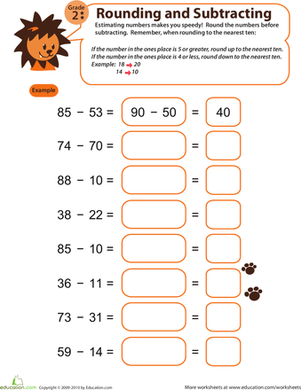Speedy Rounding and Subtracting 2

Watch your second grade's estimation and subtraction skills skyrocket with this fun rounding and subtracting worksheet!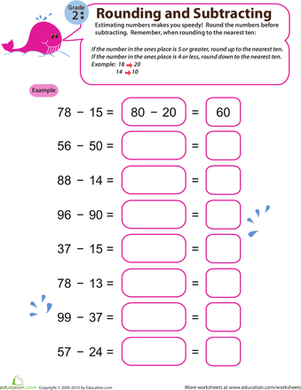Speedy Rounding and Subtracting 3

With sufficient estimation practice, your second grader will gain a better understanding of numbers and significantly improve her subtraction skills!Speedy Rounding and Subtracting 4

Sharpen your second grader's ability to subtract quickly and efficiently by integrating estimation into the process with this adorable worksheet.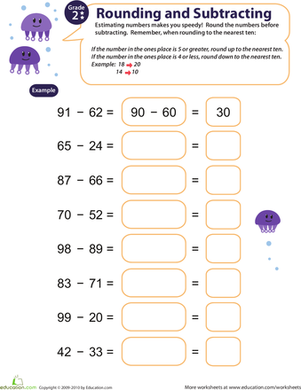Speedy Rounding and Subtracting 5

With this adorable worksheet, your second grader will gain a better understanding of numbers and learn how to estimate subtraction problems quickly.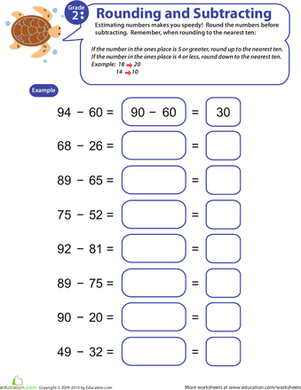Speedy Rounding and Subtracting 6

This adorable printable asks your second grader to round to the nearest ten, and then complete a speedy subtraction equation.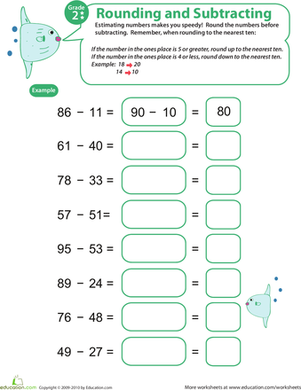Speedy Rounding and Subtracting 7

Introduce your second grader to this cute worksheet, and watch her estimation and subtraction skills skyrocket!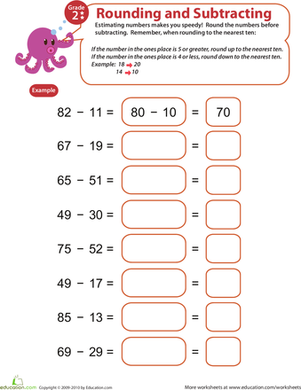Speedy Rounding and Subtracting 8

We're willing to bet that your second grader will learn how to subtract quickly and efficiently by incorporating estimation into the process!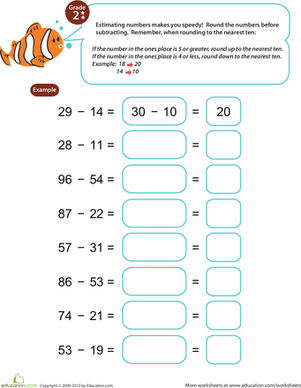Speedy Rounding and Subtracting 9

As your second grader practices working with rounded numbers, he will improve not only his subtraction, but his overall math skills as well.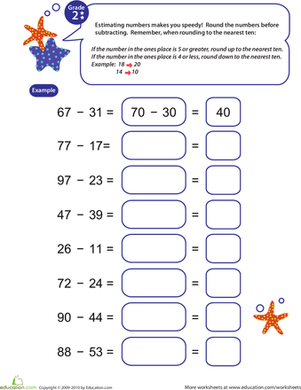Speedy Rounding and Subtracting 10

Improve your second grader's subtraction and estimation skills with this charming, colorful worksheet.

Want to download this whole set as a single PDF?

Create new collection

0

New Collection>

0 items

What could we do to improve Education.com?

Please note: Use the Contact Us link at the bottom of our website for account-specific questions or issues.

What would make you love Education.com?